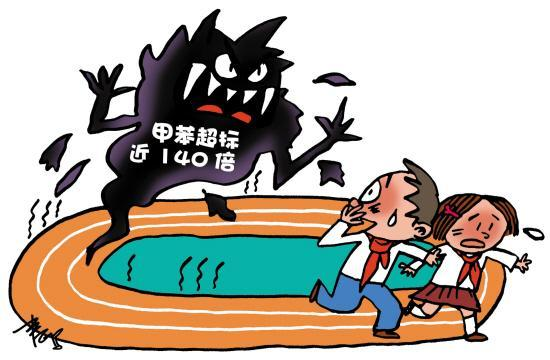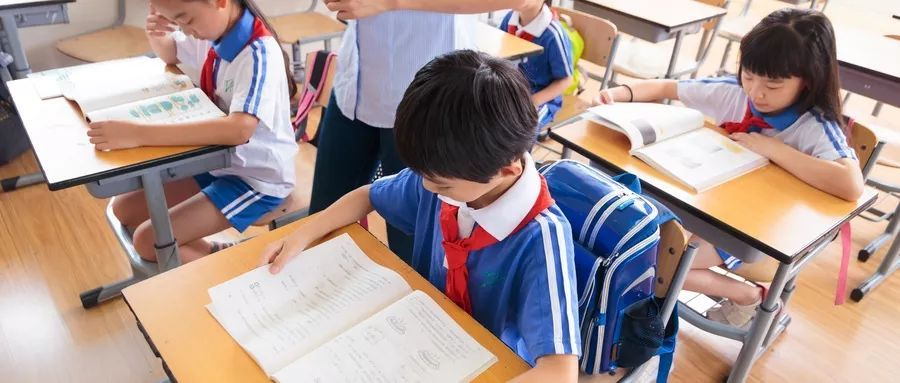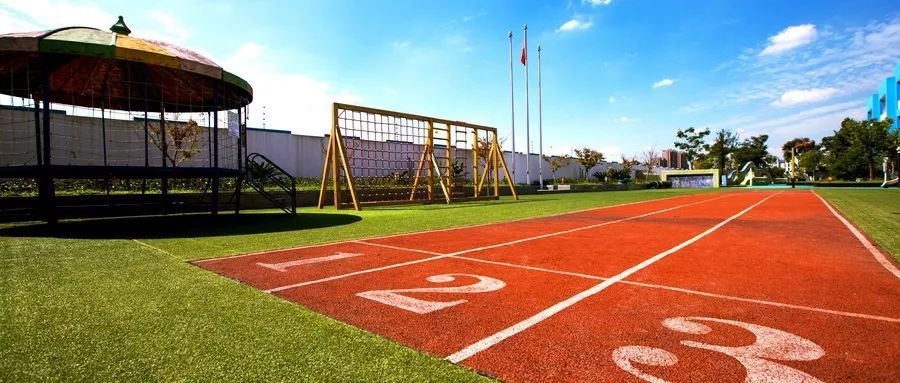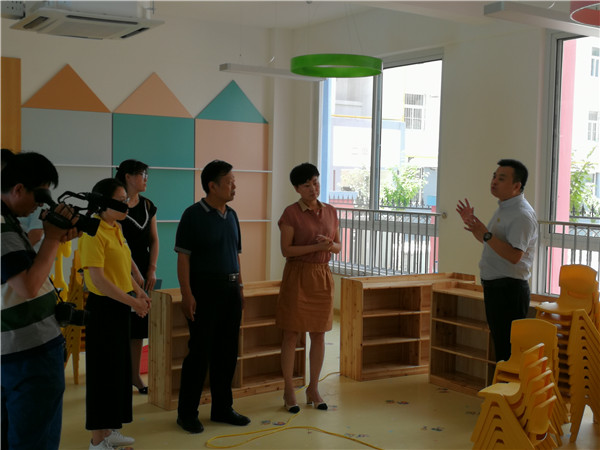## 【校园装修】学生放暑假学校装修忙 开学之后甲醛能散干净吗？<p helvetica="" neue",="" "pingfang="" sc",="" "hiragino="" sans="" gb",="" "microsoft="" yahei="" ui",="" yahei",="" arial,="" sans-serif;="" font-size:="" 17px;="" letter-spacing:="" 0.544px;="" white-space:="" normal;="" background-color:="" rgb(255,="" 255,="" 255);="" box-sizing:="" border-box="" !important;="" word-wrap:="" break-word="" !important;"="" style="margin-top: 0px; margin-bottom: 0px; padding: 0px; max-width: 100%; clear: both; min-height: 1em; color: rgb(51, 51, 51);">

• 发表评论
• 查看评论